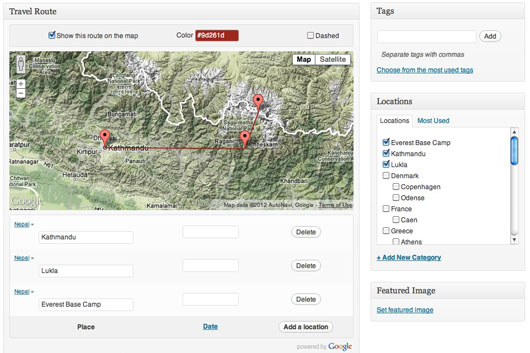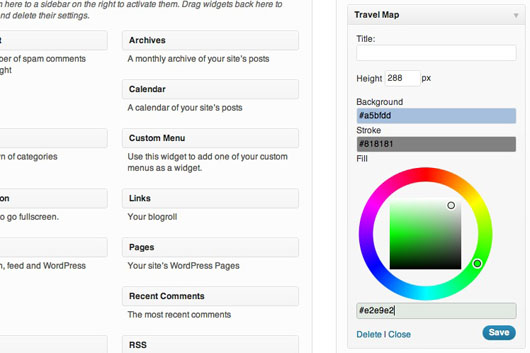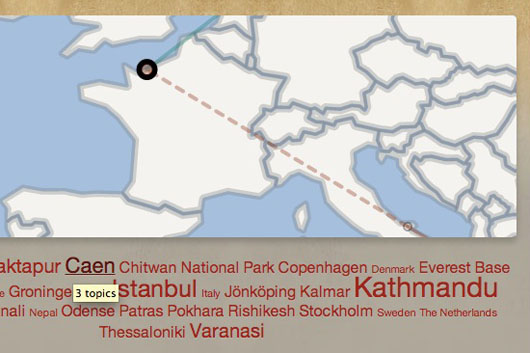## Basic Collisions Detection, RayCasting with Three.Js

I thought I would have to use a physics engine (like Cannon.Js or Ammo.Js), but Three.Js on its own is enough to sort us out with collisions, thanks to its Raycaster’s .intersectObjects() method.

This post follows a previous one, about setting up a scene and basic character controls with Three.Js, that maybe you should read if you need to understand a bit more those Character and World classes I’m playing with here.

## Using the RayCaster

Even after a little read about raycasting, I’m not quite sure I can define its concept properly… But let’s put it like this : from one origin (the very center position of our character’s Object3D), we’re going to spread vectors in every direction we’re able to move. For each one of those “rays“, we’ll be able to test if it intersects with any given mesh, and if so, to disable any move in that direction.

## Collecting the obstacles

So first of all, we need to collect every obstacle in an array : all the meshes that we’re not supposed to cross.

``````var World = Class.extend({
/* ... */
getObstacles: function () {
'use strict';
return this.obstacles.concat(this.walls);
}
});``````

## Testing and prevent collisions

Our little character motions are based on its .direction vector. Now by testing every possible direction (with the rays), we’ll be able to update that vector for it not to drive us into an obstacle.

``````var Character = Class.extend({
// Class constructor
init: function (args) {
/* ... */
// Set the character modelisation object
this.mesh = new THREE.Object3D();
/* ... */
// Set the rays : one vector for every potential direction
this.rays = [
new THREE.Vector3(0, 0, 1),
new THREE.Vector3(1, 0, 1),
new THREE.Vector3(1, 0, 0),
new THREE.Vector3(1, 0, -1),
new THREE.Vector3(0, 0, -1),
new THREE.Vector3(-1, 0, -1),
new THREE.Vector3(-1, 0, 0),
new THREE.Vector3(-1, 0, 1)
];
// And the "RayCaster", able to test for intersections
this.caster = new THREE.Raycaster();
},
// Test and avoid collisions
collision: function () {
'use strict';
var collisions, i,
// Maximum distance from the origin before we consider collision
distance = 32,
// Get the obstacles array from our world
obstacles = basicScene.world.getObstacles();
// For each ray
for (i = 0; i < this.rays.length; i += 1) {
// We reset the raycaster to this direction
this.caster.set(this.mesh.position, this.rays[i]);
// Test if we intersect with any obstacle mesh
collisions = this.caster.intersectObjects(obstacles);
// And disable that direction if we do
if (collisions.length > 0 && collisions.distance <= distance) {
// Yep, this.rays[i] gives us : 0 => up, 1 => up-left, 2 => left, ...
if ((i === 0 || i === 1 || i === 7) && this.direction.z === 1) {
this.direction.setZ(0);
} else if ((i === 3 || i === 4 || i === 5) && this.direction.z === -1) {
this.direction.setZ(0);
}
if ((i === 1 || i === 2 || i === 3) && this.direction.x === 1) {
this.direction.setX(0);
} else if ((i === 5 || i === 6 || i === 7) && this.direction.x === -1) {
this.direction.setX(0);
}
}
}
},
// Process the character motions
motion: function () {
'use strict';
// Update the directions if we intersect with an obstacle
this.collision();
// If we're not static
if (this.direction.x !== 0 || this.direction.z !== 0) {
// Rotate the character
this.rotate();
// Move the character
this.move();
return true;
}
}
/* ... */
});``````

And that’s it. So of course this isn’t perfect : some meshes are not taken into account (the hands and feet for instance), and it’s possible for some obstacles to get through our rays (try to walk just a little bit too close beside the cube on the demo). One solution would be to add some more rays. But still, this is meant to stay basic, so I’ll keep it like this so far.

See ya folks !

## Basic Character Controls with Three.Js

I wanted to try Three.Js out since a little while, and I finally found some time for it. I’ll detail here the different classes I wrote for this to work.

Notice that the collisions are not handled yet. But this will be the topic of an upcoming post, as soon as I figure it out !

I used John Resig’s Simple JavaScript Inheritance in order to get rid of those ugly prototype declarations, to get a cleaner code, and to simplify any further implementation that would require classes inherithance.

Oh, that, and Paul Irish’s requestAnimationFrame polyfill.

That’s it for the tools. You don’t have to use them, but I will in the following explanations. In case you don’t, just replace the classes declarations by their prototype equivalences.

Now the first thing to say about what I experimented : so much trigonometry fun ! Let’s go.

## Set the scene

I’m rendering within a `<figure>` tag.

``<figure id="basic-scene"></figure>``

Now we got to create the scene. We need a camera, to define from which view point we’re looking, some light or we won’t see a thing, and a “renderer”, which is actually the canvas viewport we render with.

This main class here will also set some event listeners for us to handle the user’s interactions, and a “frame” method that we’ll call at every animation frame request.

``````var basicScene;
var BasicScene = Class.extend({
// Class constructor
init: function () {
'use strict';
// Create a scene, a camera, a light and a WebGL renderer with Three.JS
this.scene = new THREE.Scene();
this.camera = new THREE.PerspectiveCamera(45, 1, 0.1, 10000);
this.light = new THREE.PointLight();
this.light.position.set(-256, 256, -256);
this.renderer = new THREE.WebGLRenderer();
// Define the container for the renderer
this.container = jQuery('#basic-scene');
// Create the user's character
this.user = new Character({
color: 0x7A43B6
});
// Create the "world" : a 3D representation of the place we'll be putting our character in
this.world = new World({
color: 0xF5F5F5
});
// Define the size of the renderer
this.setAspect();
// Insert the renderer in the container
this.container.prepend(this.renderer.domElement);
// Set the camera to look at our user's character
this.setFocus(this.user.mesh);
// Start the events handlers
this.setControls();
},
// Event handlers
setControls: function () {
'use strict';
// Within jQuery's methods, we won't be able to access "this"
var user = this.user,
// State of the different controls
controls = {
left: false,
up: false,
right: false,
down: false
};
// When the user presses a key
jQuery(document).keydown(function (e) {
var prevent = true;
// Update the state of the attached control to "true"
switch (e.keyCode) {
case 37:
controls.left = true;
break;
case 38:
controls.up = true;
break;
case 39:
controls.right = true;
break;
case 40:
controls.down = true;
break;
default:
prevent = false;
}
// Avoid the browser to react unexpectedly
if (prevent) {
e.preventDefault();
} else {
return;
}
// Update the character's direction
user.setDirection(controls);
});
// When the user releases a key
jQuery(document).keyup(function (e) {
var prevent = true;
// Update the state of the attached control to "false"
switch (e.keyCode) {
case 37:
controls.left = false;
break;
case 38:
controls.up = false;
break;
case 39:
controls.right = false;
break;
case 40:
controls.down = false;
break;
default:
prevent = false;
}
// Avoid the browser to react unexpectedly
if (prevent) {
e.preventDefault();
} else {
return;
}
// Update the character's direction
user.setDirection(controls);
});
// On resize
jQuery(window).resize(function () {
// Redefine the size of the renderer
basicScene.setAspect();
});
},
// Defining the renderer's size
setAspect: function () {
'use strict';
// Fit the container's full width
var w = this.container.width(),
// Fit the initial visible area's height
h = jQuery(window).height() - this.container.offset().top - 20;
// Update the renderer and the camera
this.renderer.setSize(w, h);
this.camera.aspect = w / h;
this.camera.updateProjectionMatrix();
},
// Updating the camera to follow and look at a given Object3D / Mesh
setFocus: function (object) {
'use strict';
this.camera.position.set(object.position.x, object.position.y + 128, object.position.z - 256);
this.camera.lookAt(object.position);
},
// Update and draw the scene
frame: function () {
'use strict';
// Run a new step of the user's motions
this.user.motion();
// Set the camera to look at our user's character
this.setFocus(this.user.mesh);
// And draw !
this.renderer.render(this.scene, this.camera);
}
});``````

## Hello World

Or so… Because of obvious performance reasons, we won’t recreate an exact 3D representation of planet earth. We’ll create one ground and four walls instead. But I’ll call it world anyway.

Using a Object3D instead of a simple Mesh allows us to update independently the geometries within this very group of meshes.

``````var World = Class.extend({
// Class constructor
init: function (args) {
'use strict';
// Set the different geometries composing the room
var ground = new THREE.PlaneGeometry(512, 1024),
height = 128,
walls = [
new THREE.PlaneGeometry(ground.height, height),
new THREE.PlaneGeometry(ground.width, height),
new THREE.PlaneGeometry(ground.height, height),
new THREE.PlaneGeometry(ground.width, height)
],
obstacles = [
new THREE.CubeGeometry(64, 64, 64)
],
// Set the material, the "skin"
material = new THREE.MeshLambertMaterial(args),
i;
// Set the "world" modelisation object
this.mesh = new THREE.Object3D();
// Set and add the ground
this.ground = new THREE.Mesh(ground, material);
this.ground.rotation.x = -Math.PI / 2;
// Set and add the walls
this.walls = [];
for (i = 0; i < walls.length; i += 1) {
this.walls[i] = new THREE.Mesh(walls[i], material);
this.walls[i].position.y = height / 2;
}
this.walls.rotation.y = -Math.PI / 2;
this.walls.position.x = ground.width / 2;
this.walls.rotation.y = Math.PI;
this.walls.position.z = ground.height / 2;
this.walls.rotation.y = Math.PI / 2;
this.walls.position.x = -ground.width / 2;
this.walls.position.z = -ground.height / 2;
// Set and add the obstacles
this.obstacles = [];
for (i = 0; i < obstacles.length; i += 1) {
this.obstacles[i] = new THREE.Mesh(obstacles[i], material);
}
this.obstacles.position.set(0, 32, 128);
}
});``````

And now the funny part…

## Live little 3D character, live !

Here as well, we use an Object3D in order to group our meshes.

Our character has two different types of motion to update : its position and its rotation. Further on, we could easily make it jump, dive, or dance. But let’s start with a simple “moving around” action only.

A direction vector will represent the motion that our user is calling through the controls.

The step property will record the progression of the character’s position motion. We will use it to animate its feet and hands.

The feet of our simple character are half-spheres. The trick is simply to properly set the phiStart, phiLength, thetaStart and thetaLength parameters of the SphereGeometry method.

``````var Character = Class.extend({
// Class constructor
init: function (args) {
'use strict';
// Set the different geometries composing the humanoid
var head = new THREE.SphereGeometry(32, 8, 8),
hand = new THREE.SphereGeometry(8, 4, 4),
foot = new THREE.SphereGeometry(16, 4, 4, 0, Math.PI * 2, 0, Math.PI / 2),
nose = new THREE.SphereGeometry(4, 4, 4),
// Set the material, the "skin"
material = new THREE.MeshLambertMaterial(args);
// Set the character modelisation object
this.mesh = new THREE.Object3D();
this.mesh.position.y = 48;
// Set and add its hands
this.hands = {
left: new THREE.Mesh(hand, material),
right: new THREE.Mesh(hand, material)
};
this.hands.left.position.x = -40;
this.hands.left.position.y = -8;
this.hands.right.position.x = 40;
this.hands.right.position.y = -8;
// Set and add its feet
this.feet = {
left: new THREE.Mesh(foot, material),
right: new THREE.Mesh(foot, material)
};
this.feet.left.position.x = -20;
this.feet.left.position.y = -48;
this.feet.left.rotation.y = Math.PI / 4;
this.feet.right.position.x = 20;
this.feet.right.position.y = -48;
this.feet.right.rotation.y = Math.PI / 4;
// Set and add its nose
this.nose = new THREE.Mesh(nose, material);
this.nose.position.y = 0;
this.nose.position.z = 32;
// Set the vector of the current motion
this.direction = new THREE.Vector3(0, 0, 0);
// Set the current animation step
this.step = 0;
},
// Update the direction of the current motion
setDirection: function (controls) {
'use strict';
// Either left or right, and either up or down (no jump or dive (on the Y axis), so far ...)
var x = controls.left ? 1 : controls.right ? -1 : 0,
y = 0,
z = controls.up ? 1 : controls.down ? -1 : 0;
this.direction.set(x, y, z);
},
// Process the character motions
motion: function () {
'use strict';
// (if any)
if (this.direction.x !== 0 || this.direction.z !== 0) {
// Rotate the character
this.rotate();
// And, only if we're not colliding with an obstacle or a wall ...
if (this.collide()) {
return false;
}
// ... we move the character
this.move();
return true;
}
},
// Rotate the character
rotate: function () {
'use strict';
// Set the direction's angle, and the difference between it and our Object3D's current rotation
var angle = Math.atan2(this.direction.x, this.direction.z),
difference = angle - this.mesh.rotation.y;
// If we're doing more than a 180°
if (Math.abs(difference) > Math.PI) {
// We proceed to a direct 360° rotation in the opposite way
if (difference > 0) {
this.mesh.rotation.y += 2 * Math.PI;
} else {
this.mesh.rotation.y -= 2 * Math.PI;
}
// And we set a new smarter (because shorter) difference
difference = angle - this.mesh.rotation.y;
// In short : we make sure not to turn "left" to go "right"
}
// Now if we haven't reached our target angle
if (difference !== 0) {
// We slightly get closer to it
this.mesh.rotation.y += difference / 4;
}
},
move: function () {
'use strict';
// We update our Object3D's position from our "direction"
this.mesh.position.x += this.direction.x * ((this.direction.z === 0) ? 4 : Math.sqrt(8));
this.mesh.position.z += this.direction.z * ((this.direction.x === 0) ? 4 : Math.sqrt(8));
// Now let's use Sine and Cosine curves, using our "step" property ...
this.step += 1 / 4;
// ... to slightly move our feet and hands
this.feet.left.position.setZ(Math.sin(this.step) * 16);
this.feet.right.position.setZ(Math.cos(this.step + (Math.PI / 2)) * 16);
this.hands.left.position.setZ(Math.cos(this.step + (Math.PI / 2)) * 8);
this.hands.right.position.setZ(Math.sin(this.step) * 8);
},
collide: function () {
'use strict';
// INSERT SOME MAGIC HERE
return false;
}
});``````

I started to look at Cannon.JS to deal with the collisions, but it will have to wait for another post.

Now let’s call our main object and start the animated rendering.

``````basicScene = new BasicScene();
function animate () {
requestAnimationFrame(animate);
basicScene.frame();
}
animate();``````

And here we are !

## Conclusion

Isn’t it surprising how easy it became to set up in-browser 3D ? Thanks Mr.doob for Three.JS !

This is only an experiment on a basic set up, but I would like to upgrade it every now and then. The next step will be the collisions detection.

Have fun fellows, I hope I’ll see you around again !

## Board Game Experiment, with JS Prototypes and CanvasOkay fellows. It took me ages, but I coded my very first game in JavaScript. It’s based on Quoridor, a really interesting “abstract strategy” board game, designed by Mirko Marchesi and published by Gigamic. The rules and logic of it are quite simple, so I thought it would be a nice project for my first experiment of this kind.

What I’m about to do here, is to review step by step how I made it. It was my first real use of Javascript Prototypes and HTML5 canvas, and I learned a lot, so maybe this little “tutorial” will be interesting for you.

The game “Quoridor” is legally owned and protected by Gigamic, so you guys understand that this code can’t go further than our private experimental use.

Maybe you would like to play the demo before we make this work and rock.

## Travel Routes WordPress Plugin

Easily add geographical tags on a map when you write a post, and it will automatically create new countries and localities terms. You can also order those locations randomly or by date to define your routes.Use the map as a widget, and pick your own colors to customize it.It is a SVG map that react to users actions (mouse over posts and terms links, click on route line…).https://wordpress.org/plugins/travel-routes/

Enjoy folks.